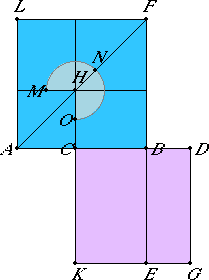# Proposition 2

If the square on a straight line is five times the square on a segment on it, then, when the double of the said segment is cut in extreme and mean ratio, the greater segment is the remaining part of the original straight line.

Let the square on the straight line AB be five times the square on the segment AC of it, and let CD be double AC.

I say that, when CD is cut in extreme and mean ratio, then the greater segment is CB.I.46

Describe the squares AF and CG on AB and CD respectively, draw the figure in AF, and draw BE through.

Now, since the square on BA is five times the square on AC, therefore AF is five times AH. Therefore the gnomon MNO is quadruple AH.

And, since DC is double CA, therefore the square on DC is quadruple the square on CA, that is, CG is quadruple AH. But the gnomon MNO is also quadruple AH, therefore the gnomon MNO equals CG.

VI.1

And, since DC is double CA, while DC equals CK, and AC equals CH, therefore KB is also double BH.

But the sum of LH and HB is also double HB, therefore KB equals the sum of LH and HB.

But the whole gnomon MNO was also proved equal to the whole CG, therefore the remainder HF equals BG.

And BG is the rectangle CD by DB, for CD equals DG, and HF is the square on CB, therefore the rectangle CD by DB equals the square on CB.

Therefore DC is to CB as CB is to BD. But DC is greater than CB, therefore CB is also greater than BD.

Therefore, when the straight line CD is cut in extreme and mean ratio, CB is the greater segment.

Q.E.D.

# Lemma

That the double AC is greater than BC is to be proved thus.

If not, let BC be, if possible, double CA.

Therefore the square on BC is quadruple the square on CA. Therefore the sum of the squares on BC and CA is five times the square on CA. But, by hypothesis, the square on BA is also five times the square on CA

II.4

Therefore the square on BA equals the sum of the squares on BC and CA, which is impossible.

Therefore CB is not double AC.

Similarly we can prove that neither is a straight line less than CB double CA, for the absurdity is much greater.

Therefore the double AC is greater than CB.

Therefore, if the square on a straight line is five times the square on a segment on it, then, when the double of the said segment is cut in extreme and mean ratio, the greater segment is the remaining part of the original straight line.

Q.E.D.

## Guide

This proposition is not used in the rest of the Elements. It is only included because it is the converse of the previous proposition XIII.1 and, thus, gives an algebraic characterization of cutting in extreme and mean ratio.